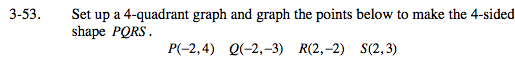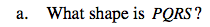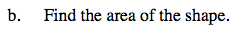### Home > MC2 > Chapter 3 > Lesson 3.1.5 > Problem3-53

3-53.Plot the points P, Q, R, and S with their respective coordinates to form the 4-sided shape.Look closely at the shape created by the points. Do you notice any special characteristics about the shape?
Did you notice that it had a pair of parallel sides?Consider the trapezoid as separate parts and visualize the parts reconstructed as a rectangle.
Try summing the area of the different pieces.

Use the formula below from Math Notes Box Lesson 3.1.1 for extra help to calculate the area of the trapezoid.

$A = \frac{1}{2} h(a + b)$

Explore the problem with the eTool below.
Click the link at right for the full version of the eTool: MC2 3-53 HW eTool